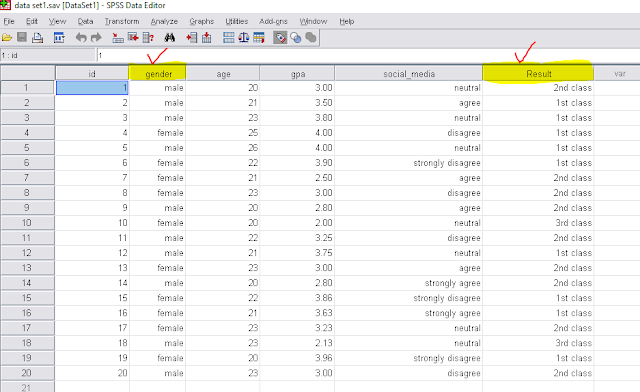# Bivariate analysis using spss (data analysis part-10)

Bivariate analysis is a statistical analysis which deals with two variables and find the relationship between the variables. We can perform bivariate analysis using SPSS, Stata and other statistical software very easily.Bivariate Analysis

## What is bivariate analysis?

Bivariate analysis is the analysis of two random variable and find their association. The casualty and association is tested using the bivariate analysis. Based on changes to an independent variable, it is possible to predict the value of a dependent variable. For bivariate analysis in SPSS software, we mainly use crosstabs and to show the association we use chi-square test.
There are some statistical techniques by which we can easily find the bivariate relationship.
• Correlation Coefficients: The coefficient shows the relationship between two variables. When the correlation coefficient is zero then this means that the variables are not related. If the correlation coefficient is a positive or a negative 1 then this means that the variables are perfectly correlated.
• Regression Analysis: It is the most widely used technique to find the bivariate relationship of two variables. It also helps to find the correlation coefficient.
• Scatter plots: This gives an idea of the patterns that can be formed using the two variables.

### Bivariate analysis using SPSS

For bivariate analysis in SPSS we use the following data set and conduct bivariate analysis for the two highlighted variable (gender and result) and we also fond their association.Data set

1. First we have to go to–

Analyze →→→Descriptive statistics →→→→CrosstabsCrosstabs

2. Now we can see two box named Row and Column and we have to enter one variable into Row box and another into column box and click on statistics.Row and column box

3. In statistics we have to select chi-square and click on continue.Chi-square

4. Finally we have to click on OK and then we the see the output window.click on OK

OUTPUT

### Interpretation:

To interpret the chi square value we have build a hypothesis for association test. Let,
H0: There is no association between gender and result, vs
H1: H0 is not true.
Here we have a p-value 0.895 which is greater than 0.05. we we can not reject our null hypothesis. That means our null hypothesis is accepted and there is no association between gender and result.
• A small p-value (typically ≤ 0.05) indicates strong evidence against the null hypothesis, so we reject the null hypothesis.
• A largep-value (> 0.05) indicates weak evidence against the null hypothesis, so we fail to reject the null hypothesis.
• p-values very close to the cutoff (0.05) are considered to be marginal.

Try some fresh contents: